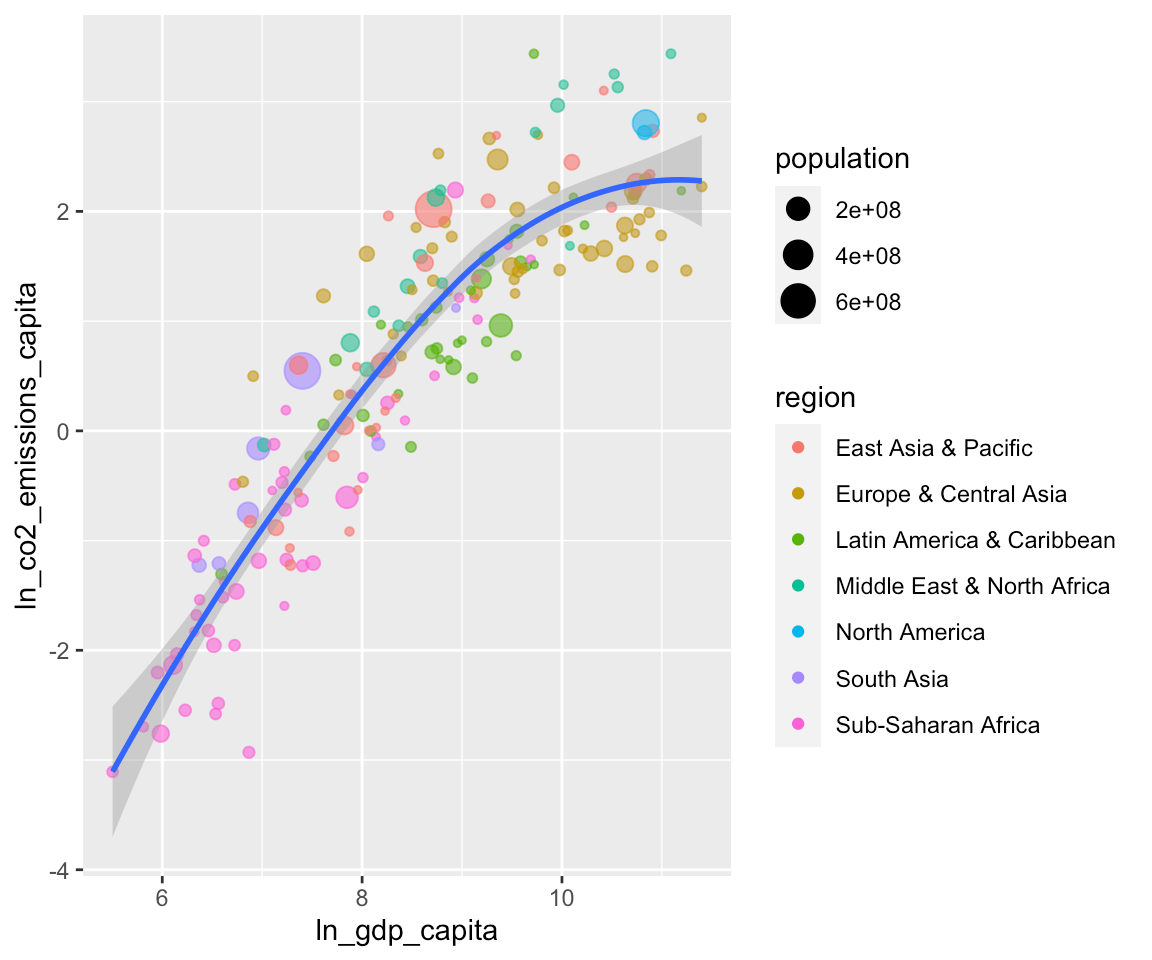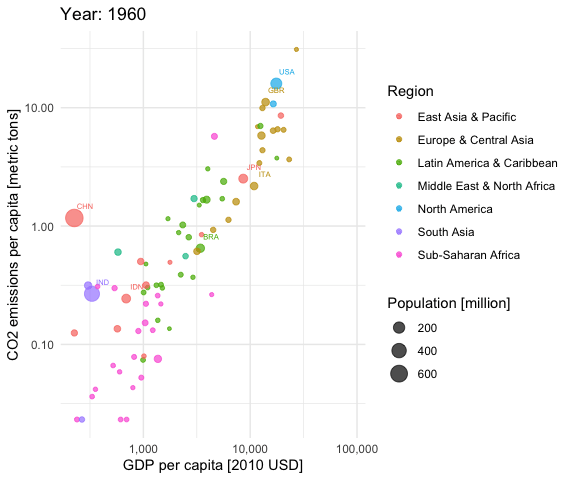[This article was first published on An Accounting and Data Science Nerd's Corner, and kindly contributed to R-bloggers]. (You can report issue about the content on this page here)
Want to share your content on R-bloggers? click here if you have a blog, or here if you don't.

Assume that you have some new data that you want to explore. The new CRAN
version of the ‘ExPanDaR’ package helps by providing a (customized) R notebook
containing all building blocks of an exploratory data analysis with a few
clicks.

## Install the Package and Start ExPanD

First, you need to install the package. I recommend installing the Github
development version of the package as it fixes some small bugs that I
discovered directly after submitting to CRAN (sigh…).

```# Install the current development version
devtools::install_github("joachim-gassen/ExPanDaR")

# Install from CRAN
# install.packages("ExPanDaR")```

Next, you start `ExPanD()`, the shiny app of the package that is designed for
interactive data exploration. When you start ExPanD with the parameter
`export_nb_option = TRUE`, it allows you to export an R notebook containing
the current state of your analysis from within the ExPanD app.

To explore country-level CO2 emissions we will use the World Bank data that
comes with the package but you can do the same with just about every data frame
that contains at least two numerical variables by simply calling
`ExPanD(data_frame, export_nb_option = TRUE)`.

```library(ExPanDaR)

ExPanD(worldbank, df_def = worldbank_data_def, var_def = worldbank_var_def,
export_nb_option = TRUE)```

After a little bit of a wait, you will see a shiny app that lets you explore
a country-year panel of World Bank data. Select some visuals to display the
distribution of CO2 emissions, measured in metric tons per inhabitant
(`cO2_emissions_capita`). You will see that the distribution is log-normal: Many
countries have relatively low levels of emissions while few countries have very
high levels. You can define a logged variant of `cO2_emissions_capita` in the
app to see that this variable is more normally distributed.

Let’s assume that at some point, you are done interactively exploring the data
and want to export your findings for future study. One thing that you can do is
that you can save your current app choices (scroll down, hit save). For the
lazy: The code below reads my choices and starts ExPanD with them so that you
can follow the analysis below (the even lazier can also just access the shiny
app online here).

```config_co2 <- readRDS(url("https://joachim-gassen.github.io/data/ExPanD_config_co2.RDS"))

ExPanD(worldbank, df_def = worldbank_data_def,
var_def = worldbank_var_def,
config_list = config_co2, export_nb_option = TRUE)```

## Export a Notebook Containing Your Analysis

While this allows you to restart ExPanD with your current analysis at a later
point, you most likely want to extend the analysis by hand, e.g. by estimating
more refined models or by adding more visuals. For this, you can export a
Notebook containing the analysis. To do so, scroll to the bottom of the ExPanD
app and click on the button below.named `ExPanD_nb.zip`. Store and unzip it wherever you like. It contains two
files

• A notebook file `ExPanD_nb_code.Rmd` and
• a data file `ExPanD_nb_data.RData` containing data and variable definitions.

## Exploring the Notebook Code

Use RStudio to open the notebook file. You can directly knit it
(`Preview/Knit to HTML`) but in order to work with and extend it, it is useful
to take a deeper look at its code first. The new vignette
of the package contains more detailed information on the Notebook code. In this
blog post, I will focus on an example for how to extend the analysis.

When you scroll down the notebook, you will see the chunk that creates the
scatter plot. In my analysis, I used it to document that the log of CO2
emissions per capita is almost perfectly proportional to the log of GDP per
capita. See below:

```df <- smp
df <- df[df\$year == "2014", ]
df <- df[, c("country", "year", "ln_gdp_capita",
"ln_co2_emissions_capita", "region", "population")]
df <- df[complete.cases(df), ]
df\$region <- as.factor(df\$region)
prepare_scatter_plot(df, "ln_gdp_capita", "ln_co2_emissions_capita",
color = "region", size = "population", loess = 1)```You see that the code of the chunk uses `prepare_scatter_plot()` of the
‘ExPanDaR’ package to quickly produce a scatter plot visualizing up to four data
dimensions. See the help pages of the package and the vignette “Using the
functions of the ExPanDaR package”

for more information on how to use the EDA functions that come with the package.
Also, you can always take a look at their code (just call their name without the
brackets) to see what they do under the hood and to extend or modify them.

## Modifying and Extending the Notebook

The finding above makes intuitive sense. Economic activity uses resources. Thus,
we would expect countries with higher levels of economic activity to generate
higher levels of CO2 emissions. As you can see above, the analysis is based on
observations from 2014. Based on our argument above, the relation should also
hold over longer periods. Let’s see how it looks like when we look at 1980. To
do so, just copy and paste the chunk above and change the sample screen to be
`"1980"`.

```df <- smp
df <- df[df\$year == "1980", ]
df <- df[, c("country", "year", "ln_gdp_capita",
"ln_co2_emissions_capita", "region", "population")]
df <- df[complete.cases(df), ]
df\$region <- as.factor(df\$region)
prepare_scatter_plot(df, "ln_gdp_capita", "ln_co2_emissions_capita",
color = "region", size = "population", loess = 1)```Seems to be the case. How does it look when we use all data (just remove the
screen)?

```df <- smp
df <- df[, c("country", "year", "ln_gdp_capita",
"ln_co2_emissions_capita", "region", "population")]
df <- df[complete.cases(df), ]
df\$region <- as.factor(df\$region)
prepare_scatter_plot(df, "ln_gdp_capita", "ln_co2_emissions_capita",
color = "region", size = "population", loess = 1)```Looking at the whole data frame, the trajectories of the individual countries
become apparent. The big red caterpillar to the left is China, the purple one
below that is India and the Blue blob in the upper right is the U.S. This calls
for a niced-up “Hans-Rosling-style” animation. Let’s do this.

```library(gganimate)

iso3c <- worldbank %>% select(iso3c, country) %>% distinct()
df <- smp %>% left_join(iso3c, by = "country")
df <- df[, c("iso3c", "country", "year", "gdp_capita",
"co2_emissions_capita", "region", "population")]
df <- df[complete.cases(df),] %>%
filter(year <= 2014) %>%
group_by(country) %>%
mutate(nobs = n()) %>%
ungroup() %>%
filter(nobs == max(nobs))

df\$region <- as.factor(df\$region)
df\$year <- as.integer(as.character(df\$year))
df\$population <- df\$population / 1e6

aplot <- ggplot(df, aes(gdp_capita, co2_emissions_capita,
size = population, colour = region)) +
geom_point(alpha = 0.7) +
scale_x_log10(labels = scales::comma) +
scale_y_log10(labels = scales::comma) +
scale_size_continuous(labels = scales::comma) +
labs(title = 'Year: {frame_time}',
x = 'GDP per capita [2010 USD]',
y = "CO2 emissions per capita [metric tons]",
color = "Region",
size = "Population [million]") +
theme_minimal() +
geom_text(data = subset(df, population > 50), aes(label = iso3c),
nudge_x = 0.1, nudge_y = 0.1, size = 2) +
transition_time(year) +
ease_aes('linear')

animate(aplot, start_pause = 20, end_pause = 20)```You see that most of the countries are moving to the upper right over time. This
is good (higher economic productivity) and bad (higher CO2 emissions per capita)
at the same time. Only very few European countries seem to be moving slightly
downwards in later years, indicating reducing CO2 emissions per capita.
It is a pity that the CO2 data provided by the World Bank currently stops in 2014.

Anyhow, you see that a notebook generated with a few clicks based on the interactive
ExPanD analysis can serve as a starting point for a more in-depth analysis.
Now it is your turn. Feel free to modify and extend your analysis along all
possible dimensions. Code away and enjoy!